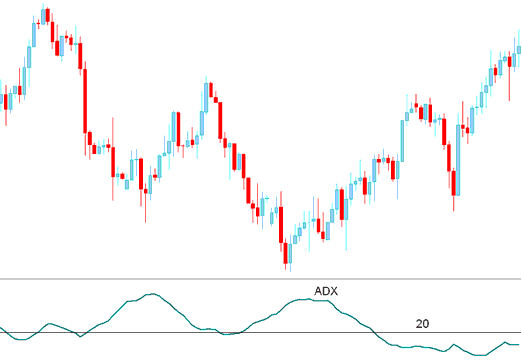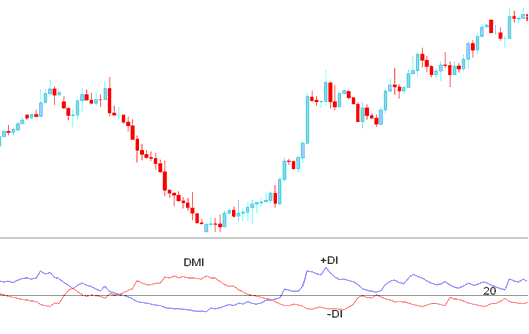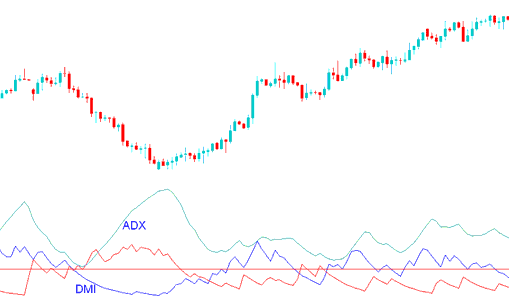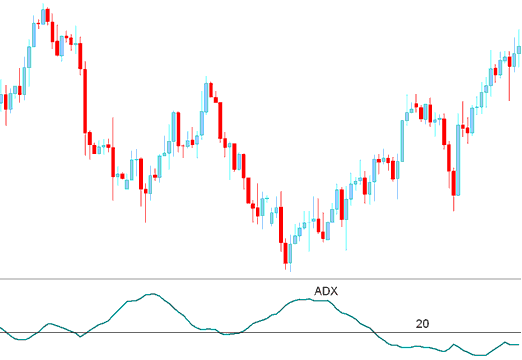Developed by J. Welles Wilder

This a momentum indicator used to determine the strength of a indices price trend; it is derived from the DMI - Directional Movement Index which has two lines.

+DI - Positive Directional Movement Index

–DI - Negative Directional Movement Index

ADX is calculated by subtracting these two values and applying a smoothing function.The ADX is not a directional indicator but a measure of the strength of the indices trend which has a scale of Zero to 100.

The higher the indicator value the stronger the trend.

A value of below 20 indicates that the stock indexes trading market is not trending but moving in a range.

A value of above 20 confirms a buy or sell stock indices signal and indicates a new indices trend is emerging.

Values above 30 signifies a strong trending market.

When ADX turns down from above 30, it signifies that the current indices trend is losing momentum.

Since the ADX alone is a directionless indicator it is combined with the DMI index to determine the direction of indices.DMIWhen the ADX is combined with DMI index a indices trader can determine the direction of the indices trend and then use the this indicator to determine the momentum of the prevailing indices trend direction.## Indices Technical Analysis and Generating Indices Trading Signals

A buy stock indices signal is generated when the +DI is above –DI, and the ADX is above 20

The Exit signal is generated when the indicator turns down from above 30.A short signal is generated when the –DI is above +DI, and the ADX is above 20

The Exit signal is generated when the indicator turns down from above 30.